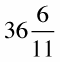##### Algebra I For DummiesChanging improper fractions to mixed numbers can help you better understand the result of an algebraic problem. Improper fractions are simply top-heavy fractions whose numerators (the numbers on top) are bigger than their denominators. Mixed numbers contain both a whole number and a fraction.

Example: Change the improper fraction 402/11 to a mixed number.

1. Divide the numerator by the denominator.

Divide 402 by 11, which equals 36 with a remainder of 6.

402 11 = 36 r6

2. Find the whole number.

The whole number is the number of times the denominator divides into the numerator.

11 (the denominator) divides into 402 (the numerator) 36 times, so 36 is the whole number.

3. Make the remainder the new numerator.

Because the remainder (the leftover value) of dividing 402 by 11 is 6, 6 is the new numerator. The denominator is still 11. So, the mixed fraction is:This mixed fraction is much easier to understand!